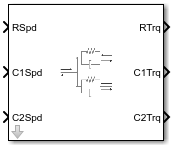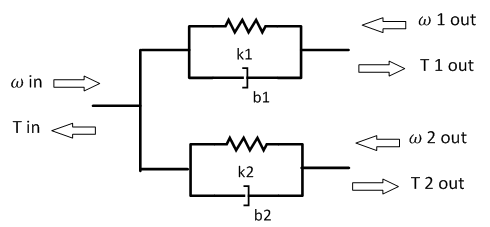Split Torsional Compliance

Split torsional coupler

• Library:
• Powertrain Blockset / Drivetrain / Couplings

Vehicle Dynamics Blockset / Powertrain / Drivetrain / Couplings

•Description

The Split Torsional Compliance block implements parallel spring-damper coupling between shafts. You can specify the type of coupling by selecting one of the Coupling Configuration parameters:

• Shaft split — Single input shaft coupled to two output shafts

• Shaft merge — Two input shafts coupled to a single output shaft

In fuel economy and emissions studies, you can use the Split Torsional Compliance block to model mechanical rotational compliance between common driveline elements such as motors, planetary gears, and clutches. For example, use the Shaft split configuration to couple a motor and two planetary gear sets. Use the Shaft merge configuration to couple a dual clutch transmission to an output shaft.

Shaft Split

For the Shaft split configuration, the block implements this schematic and equations.$\begin{array}{l}{T}_{in}=-\left({\omega }_{in}-{\omega }_{1out}\right){b}_{1}-\left({\omega }_{in}-{\omega }_{2out}\right){b}_{2}-{\theta }_{1}{k}_{1}-{\theta }_{2}{k}_{2}\\ {T}_{1out}=\left({\omega }_{in}-{\omega }_{1out}\right){b}_{1}+{\theta }_{1}{k}_{1}\\ {T}_{2out}=\left({\omega }_{in}-{\omega }_{2out}\right){b}_{2}+{\theta }_{2}{k}_{2}\\ \\ {\stackrel{˙}{\theta }}_{1}=\left({\omega }_{in}-{\omega }_{1out}\right)\\ {\stackrel{˙}{\theta }}_{2}=\left({\omega }_{in}-{\omega }_{2out}\right)\end{array}$

To account for frequency-dependent damping, both damping terms incorporate a low-pass filter.

The equations use these variables.

 Tin Resulting applied input reaction torque ωin Input shaft rotational velocity T1out Resulting applied torque to first output shaft ω1out First output shaft rotational velocity T2out Resulting applied torque to second output shaft ω2out Second output shaft rotational velocity θ1, θ2 First, second shaft rotation, respectively b1, b2 First, second shaft viscous damping, respectively k1, k2 First, second shaft torsional stiffness, respectively

Shaft Merge

For the Shaft merge configuration, the block implements this schematic and equations.$\begin{array}{l}{T}_{out}=\left(-{\omega }_{out}+{\omega }_{1in}\right){b}_{1}+\left(-{\omega }_{out}+{\omega }_{2in}\right){b}_{2}+{\theta }_{1}{k}_{1}+{\theta }_{2}{k}_{2}\\ {T}_{1out}=\left({\omega }_{out}-{\omega }_{1in}\right){b}_{1}-{\theta }_{1}{k}_{1}\\ {T}_{2out}=\left({\omega }_{out}-{\omega }_{2in}\right){b}_{2}-{\theta }_{2}{k}_{2}\\ \\ {\stackrel{˙}{\theta }}_{1}=\left({\omega }_{1in}-{\omega }_{out}\right)\\ {\stackrel{˙}{\theta }}_{2}=\left({\omega }_{2in}-{\omega }_{out}\right)\end{array}$

To account for frequency-dependent damping, both damping terms incorporate a low-pass filter.

The equations use these variables.

 Tout Resulting applied output torque ωout Output shaft rotational velocity T1in Resulting reaction torque to first input shaft ω1in First input shaft rotational velocity T2in Resulting reaction torque to second input shaft ω2in Second input shaft rotational velocity θ1, θ2 First, second shaft rotation, respectively b1, b2 First, second shaft viscous damping, respectively k1, k2 First, second shaft torsional stiffness, respectively

Power Accounting

For the power accounting, the block implements these equations.

Bus Signal DescriptionVariableEquations

PwrInfo

PwrTrnsfrd — Power transferred between blocks

• Positive signals indicate flow into block

• Negative signals indicate flow out of block

PwrR

For the Shaft split configuration, mechanical power from input shaft

PTR

PwrC1

For the Shaft split configuration, mechanical power from first output shaft

PTC1

PwrC2

For the Shaft split configuration, mechanical power from second output shaft

PTC2

PwrC

For the Shaft merge configuration, mechanical power from output shaft

PTC

PwrR1

For the Shaft merge configuration, mechanical power from first input shaft

PTR1

PwrR2

For the Shaft merge configuration, mechanical power from second input shaft

PTR2

PwrNotTrnsfrd — Power crossing the block boundary, but not transferred

• Positive signals indicate an input

• Negative signals indicate a loss

PwrDampLossMechanical damping loss

Pd

${P}_{d}=-\left({b}_{1}{|{\stackrel{˙}{\theta }}_{1}|}^{2}+{b}_{2}{|{\stackrel{˙}{\theta }}_{2}|}^{2}\right)$

PwrStored — Stored energy rate of change

• Positive signals indicate an increase

• Negative signals indicate a decrease

PwrStoredShft

Rate change in spring energy

Ps

${P}_{s}=\left({k}_{1}{\theta }_{1}{\stackrel{˙}{\theta }}_{1}+{k}_{2}{\theta }_{2}{\stackrel{˙}{\theta }}_{2}\right)$

The equations use these variables.

 TR Shaft R torque TC Shaft C torque ωR Shaft R angular velocity ωC Shaft C angular velocity θ Coupled shaft rotation k Shaft torsional stiffness b Rotational viscous damping Pt Total mechanical power Pd Power loss due to damping Ps Rate change of stored spring energy

Ports

Input

expand all

Input shaft rotational velocity, ωin, in rad/s.

Dependencies

To enable this port, set both of these parameters:

• Coupling Configuration to Shaft split

First output shaft rotational velocity, ω1out, in rad/s.

Dependencies

To enable this port, set both of these parameters:

• Coupling Configuration to Shaft split

Second output shaft rotational velocity, ω2out, in rad/s.

Dependencies

To enable this port, set both of these parameters:

• Coupling Configuration to Shaft split

Output shaft rotational velocity, ωout, in rad/s.

Dependencies

To enable this port, set both of these parameters:

• Coupling Configuration to Shaft merge

First input shaft rotational velocity, ω1in, in rad/s.

Dependencies

To enable this port, set both of these parameters:

• Coupling Configuration to Shaft merge

Second input shaft rotational velocity, ω2in, in rad/s.

Dependencies

To enable this port, set both of these parameters:

• Coupling Configuration to Shaft merge

Input shaft angular velocity, ωin, in rad/s and torque, Tin, in N·m.

Dependencies

To enable this port, select:

• Port Configuration>Two-way connection

• Coupling Configuration>Shaft split

First input shaft angular velocity, ω1in, in rad/s and torque, T1in, in N·m.

Dependencies

To enable this port, select:

• Port Configuration>Two-way connection

• Coupling Configuration>Shaft merge

Second input shaft angular velocity, ω2in, in rad/s and torque, T2in, in N·m.

Dependencies

To enable this port, select:

• Port Configuration>Two-way connection

• Coupling Configuration>Shaft merge

Output

expand all

If you set Coupling Configuration to Shaft split, the Info bus contains these signals.

SignalDescriptionVariableUnits
Trq

R

Input shaft torque

TinN·m

C1

First output shaft torque

T1outN·m

C2

Second output shaft torque

T2outN·m

Damp

C1

First output shaft damping torque

b1ω1out

N·m

C2

Second output shaft damping torque

b2ω2out

N·m

Spring

C1

First output shaft spring torque

k1θ1

N·m

C2

Second output shaft spring torque

k2θ2

N·m

Spd

R

Input shaft angular velocity

C1

First output shaft angular velocity

C2

Second output shaft angular velocity

Difference in input and first output shaft angular velocity

${\stackrel{˙}{\theta }}_{1}$

Difference in input and second output shaft angular velocity

${\stackrel{˙}{\theta }}_{2}$

PwrInfo

PwrTrnsfrd

PwrR

Mechanical power from input shaft

PTR

W
PwrC1

Mechanical power from first output shaft

PTC1

W
PwrC2

Mechanical power from second output shaft

PTC2W

PwrNotTrnsfrd

PwrDampLoss

Mechanical damping loss

Pd

W

PwrStored

PwrStoredShft

Rate change of stored internal torsional energy

Ps

W

If you set Coupling Configuration to Shaft merge, the Info bus contains these signals.

SignalDescriptionVariableUnits
Trq

C

Output shaft torque

ToutN·m

R1

First input shaft torque

T1inN·m

R2

Second input shaft torque

T2inN·m

Damp

R1

First input shaft damping torque

b1ω1in

N·m

R2

Second in shaft damping torque

b2ω2in

N·m

Spring

R1

First input shaft spring torque

k1θ1

N·m

R2

Second in shaft spring torque

k2θ2

N·m

Spd

C

Output shaft angular velocity

R1

First input shaft angular velocity

R2

Second input shaft angular velocity

Difference in first input and output shaft angular velocity

${\stackrel{˙}{\theta }}_{1}$

Difference in second input and output shaft angular velocity

${\stackrel{˙}{\theta }}_{2}$

PwrInfo

PwrTrnsfrd

PwrC

Mechanical power from output shaft

PTC

W
PwrR1

Mechanical power from first input shaft

PTR1

W
PwrR2

Mechanical power from second input shaft

PTR2W

PwrNotTrnsfrd

PwrDampLoss

Mechanical damping loss

Pd

W

PwrStored

PwrStoredShft

Rate change of stored internal torsional energy

Ps

W

Dependencies

To enable this port, select Output Info bus.

Input shaft torque, Tin, in N·m.

Dependencies

To enable this port, set both of these parameters:

• Coupling Configuration to Shaft split

First output shaft torque, T1out, in N·m.

Dependencies

To enable this port, set both of these parameters:

• Coupling Configuration to Shaft split

Second output shaft torque, T2out, in N·m.

Dependencies

To enable this port, set both of these parameters:

• Coupling Configuration to Shaft split

Output shaft torque, Tout, in N·m.

Dependencies

To enable this port, set both of these parameters:

• Coupling Configuration to Shaft merge

First input shaft torque, T1in, in N·m.

Dependencies

To enable this port, set both of these parameters:

• Coupling Configuration to Shaft merge

Second input shaft torque, T2in, in N·m.

Dependencies

To enable this port, set both of these parameters:

• Coupling Configuration to Shaft merge

First output shaft angular velocity, ω1out, in rad/s and torque, T1out, in N·m.

Dependencies

To enable this port, select:

• Port Configuration>Two-way connection

• Coupling Configuration>Shaft split

Second output shaft angular velocity, ω2out, in rad/s and torque, T2out, in N·m.

Dependencies

To enable this port, select:

• Port Configuration>Two-way connection

• Coupling Configuration>Shaft split

Output shaft angular velocity, ωout, in rad/s and torque, Tout, in N·m.

Dependencies

To enable this port, select:

• Port Configuration>Two-way connection

• Coupling Configuration>Shaft merge

Parameters

expand all

Block Options

Specify the port configuration.

Specify the coupling type.

Select to create the Info output port.

Coupling 1

Torsional damping, b1, in N·m· s/rad.

Coupling 2

Torsional damping, b2, in N·m· s/rad.

Extended Capabilities

C/C++ Code GenerationGenerate C and C++ code using Simulink® Coder™.

Introduced in R2017b

Powertrain Blockset DocumentationGet trial now